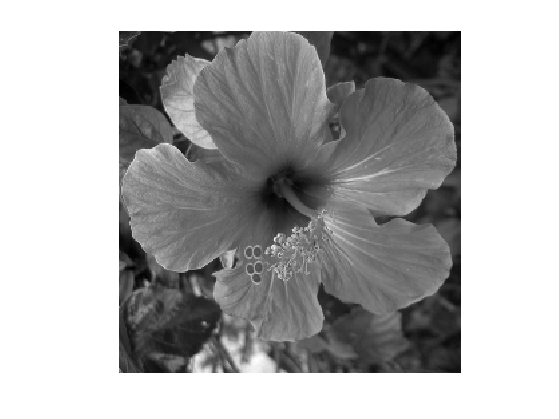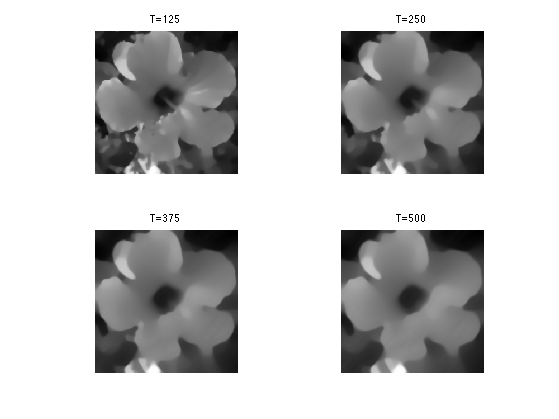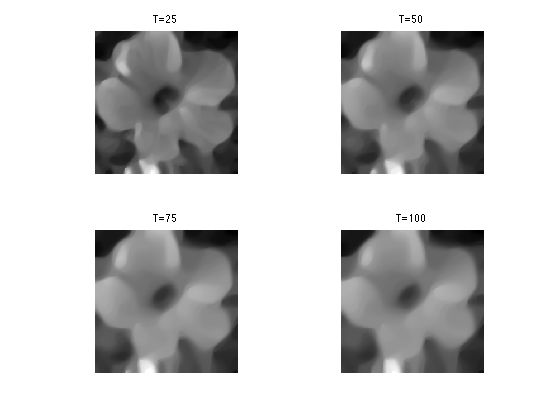$\newcommand{\NN}{\mathbb{N}} \newcommand{\CC}{\mathbb{C}} \newcommand{\GG}{\mathbb{G}} \newcommand{\LL}{\mathbb{L}} \newcommand{\PP}{\mathbb{P}} \newcommand{\QQ}{\mathbb{Q}} \newcommand{\RR}{\mathbb{R}} \newcommand{\VV}{\mathbb{V}} \newcommand{\ZZ}{\mathbb{Z}} \newcommand{\FF}{\mathbb{F}} \newcommand{\KK}{\mathbb{K}} \newcommand{\UU}{\mathbb{U}} \newcommand{\EE}{\mathbb{E}} \newcommand{\Aa}{\mathcal{A}} \newcommand{\Bb}{\mathcal{B}} \newcommand{\Cc}{\mathcal{C}} \newcommand{\Dd}{\mathcal{D}} \newcommand{\Ee}{\mathcal{E}} \newcommand{\Ff}{\mathcal{F}} \newcommand{\Gg}{\mathcal{G}} \newcommand{\Hh}{\mathcal{H}} \newcommand{\Ii}{\mathcal{I}} \newcommand{\Jj}{\mathcal{J}} \newcommand{\Kk}{\mathcal{K}} \newcommand{\Ll}{\mathcal{L}} \newcommand{\Mm}{\mathcal{M}} \newcommand{\Nn}{\mathcal{N}} \newcommand{\Oo}{\mathcal{O}} \newcommand{\Pp}{\mathcal{P}} \newcommand{\Qq}{\mathcal{Q}} \newcommand{\Rr}{\mathcal{R}} \newcommand{\Ss}{\mathcal{S}} \newcommand{\Tt}{\mathcal{T}} \newcommand{\Uu}{\mathcal{U}} \newcommand{\Vv}{\mathcal{V}} \newcommand{\Ww}{\mathcal{W}} \newcommand{\Xx}{\mathcal{X}} \newcommand{\Yy}{\mathcal{Y}} \newcommand{\Zz}{\mathcal{Z}} \newcommand{\al}{\alpha} \newcommand{\la}{\lambda} \newcommand{\ga}{\gamma} \newcommand{\Ga}{\Gamma} \newcommand{\La}{\Lambda} \newcommand{\Si}{\Sigma} \newcommand{\si}{\sigma} \newcommand{\be}{\beta} \newcommand{\de}{\delta} \newcommand{\De}{\Delta} \renewcommand{\phi}{\varphi} \renewcommand{\th}{\theta} \newcommand{\om}{\omega} \newcommand{\Om}{\Omega} \renewcommand{\epsilon}{\varepsilon} \newcommand{\Calpha}{\mathrm{C}^\al} \newcommand{\Cbeta}{\mathrm{C}^\be} \newcommand{\Cal}{\text{C}^\al} \newcommand{\Cdeux}{\text{C}^{2}} \newcommand{\Cun}{\text{C}^{1}} \newcommand{\Calt}{\text{C}^{#1}} \newcommand{\lun}{\ell^1} \newcommand{\ldeux}{\ell^2} \newcommand{\linf}{\ell^\infty} \newcommand{\ldeuxj}{{\ldeux_j}} \newcommand{\Lun}{\text{\upshape L}^1} \newcommand{\Ldeux}{\text{\upshape L}^2} \newcommand{\Lp}{\text{\upshape L}^p} \newcommand{\Lq}{\text{\upshape L}^q} \newcommand{\Linf}{\text{\upshape L}^\infty} \newcommand{\lzero}{\ell^0} \newcommand{\lp}{\ell^p} \renewcommand{\d}{\ins{d}} \newcommand{\Grad}{\text{Grad}} \newcommand{\grad}{\text{grad}} \renewcommand{\div}{\text{div}} \newcommand{\diag}{\text{diag}} \newcommand{\pd}{ \frac{ \partial #1}{\partial #2} } \newcommand{\pdd}{ \frac{ \partial^2 #1}{\partial #2^2} } \newcommand{\dotp}{\langle #1,\,#2\rangle} \newcommand{\norm}{|\!| #1 |\!|} \newcommand{\normi}{\norm{#1}_{\infty}} \newcommand{\normu}{\norm{#1}_{1}} \newcommand{\normz}{\norm{#1}_{0}} \newcommand{\abs}{\vert #1 \vert} \newcommand{\argmin}{\text{argmin}} \newcommand{\argmax}{\text{argmax}} \newcommand{\uargmin}{\underset{#1}{\argmin}\;} \newcommand{\uargmax}{\underset{#1}{\argmax}\;} \newcommand{\umin}{\underset{#1}{\min}\;} \newcommand{\umax}{\underset{#1}{\max}\;} \newcommand{\pa}{\left( #1 \right)} \newcommand{\choice}{ \left\{ \begin{array}{l} #1 \end{array} \right. } \newcommand{\enscond}{ \left\{ #1 \;:\; #2 \right\} } \newcommand{\qandq}{ \quad \text{and} \quad } \newcommand{\qqandqq}{ \qquad \text{and} \qquad } \newcommand{\qifq}{ \quad \text{if} \quad } \newcommand{\qqifqq}{ \qquad \text{if} \qquad } \newcommand{\qwhereq}{ \quad \text{where} \quad } \newcommand{\qqwhereqq}{ \qquad \text{where} \qquad } \newcommand{\qwithq}{ \quad \text{with} \quad } \newcommand{\qqwithqq}{ \qquad \text{with} \qquad } \newcommand{\qforq}{ \quad \text{for} \quad } \newcommand{\qqforqq}{ \qquad \text{for} \qquad } \newcommand{\qqsinceqq}{ \qquad \text{since} \qquad } \newcommand{\qsinceq}{ \quad \text{since} \quad } \newcommand{\qarrq}{\quad\Longrightarrow\quad} \newcommand{\qqarrqq}{\quad\Longrightarrow\quad} \newcommand{\qiffq}{\quad\Longleftrightarrow\quad} \newcommand{\qqiffqq}{\qquad\Longleftrightarrow\qquad} \newcommand{\qsubjq}{ \quad \text{subject to} \quad } \newcommand{\qqsubjqq}{ \qquad \text{subject to} \qquad }$

Non-Linear Diffusion Flows

# Non-Linear Diffusion Flows

This tours details non-linear diffusion PDEs. A good reference for diffusion flows in image processing is [Weickert98].

## Installing toolboxes and setting up the path.

You need to download the following files: signal toolbox and general toolbox.

You need to unzip these toolboxes in your working directory, so that you have toolbox_signal and toolbox_general in your directory.

For Scilab user: you must replace the Matlab comment '%' by its Scilab counterpart '//'.

Recommandation: You should create a text file named for instance numericaltour.sce (in Scilab) or numericaltour.m (in Matlab) to write all the Scilab/Matlab command you want to execute. Then, simply run exec('numericaltour.sce'); (in Scilab) or numericaltour; (in Matlab) to run the commands.

Execute this line only if you are using Matlab.

getd = @(p)path(p,path); % scilab users must *not* execute this


Then you can add the toolboxes to the path.

getd('toolbox_signal/');
getd('toolbox_general/');


## Non-linear Second-order Parabolic PDEs

This tour defines PDE flows that are non-linear extension of the heat equation. Non-linearity is crucial to produce edge-aware flows that do not blur the edges.

It is also important to produce contrast invariant and affine invariant flows.

These flows can be written as $\pd{f_t}{t}(x) = G(\nabla f_t(x), \nabla^2 f_t(x)).$ with $$f_0$$ defined at time $$t=0$$ (whith the slight modification that a blurring is introduced in the Perona-Malick flow to stabilize it).

They are discretized in space by considering a discrete image of $$N = n \times n$$ pixels.

n = 256;


We use finite difference operators $$\nabla$$ and $$\text{div}=-\nabla^*$$ with periodic boundary conditions.

Load an image $$f_0 \in \RR^N$$, that will be used to initialize the flow at time $$t=0$$.

name = 'hibiscus';
f0 = rescale( sum(f0,3) );


Display it.

clf;
imageplot(f0);The flow is discretized in time using an explicit time-stepping $f^{(\ell+1)}(x) = f^{(\ell)}(x) + \tau G(\nabla f^{(\ell)}(x), \nabla^2 f^{(\ell)}(x)).$ Here $$\tau>0$$ should be small enough, and $$f^{(\ell)}$$ produces an approximation of $$f_t$$ at time $$t=\ell\tau$$.

Convolutions can be computed in $$O(N\log(N))$$ operations using the FFT, since $g = f \star h \qarrq \forall \om, \quad \hat g(\om) = \hat f(\om) \hat h(\om).$

cconv = @(f,h)real(ifft2(fft2(f).*fft2(h)));


Define a Gaussian blurring kernel of width $$\si$$: $h_\si(x) = \frac{1}{Z} e^{ -\frac{x_1^2+x_2^2}{2\si^2} }$ where $$Z$$ ensures that $$\hat h_\si(0)=1$$.

t = [0:n/2 -n/2+1:-1];
[X2,X1] = meshgrid(t,t);
normalize = @(h)h/sum(h(:));
h = @(sigma)normalize( exp( -(X1.^2+X2.^2)/(2*sigma^2) ) );


Set the value of $$\sigma>0$$.

sigma = .5;


Define blurring operator.

blur = @(f)cconv(f,h(sigma));


## Perona-Malik Flow

The Perona-Malik non-linear diffusion is defined as $\pd{f_t}{t} = \text{div}\pa{ g_\la( \norm{\nabla f_t^\si} ) \nabla f_t }$ where $$f^\si = f \star h_\si$$.

This PDE was introduced in [PerMal90].

Here, $$g_\la : \RR^+ \rightarrow \RR^+$$ is a non-increasing function, that wechose in the following as $g_\la(s) = \frac{1}{\sqrt{1 + (s/\la)^2}}.$

g = @(s,lambda)1./sqrt( 1+(s/lambda).^2 );


Note that in the limit $$\la \rightarrow +\infty$$, one recovers the linear heat equation $\pd{f_t}{t} = \Delta f_t$ where $$\Delta=\text{div} \circ \nabla$$ is the Laplacian.

Define $$A(f) = \norm{\nabla f^\si}$$.

amplitude = @(u)repmat( sqrt( sum(u.^2,3) ), [1 1 2]);


Initialize the solution at time $$t=0$$.

f = f0;


Set the value of $$\lambda$$.

lambda = .01;


Set the value of the descent step size $$\tau>0$$.

tau = .2;


Perform one time stepping.

f = f + tau * div( g(A(f),lambda) .* grad(f) );


Final time.

T = .5/lambda;


Number of iteration to reach this final time.

niter = ceil(T/tau);


Exercice 1: (check the solution) Implement the Perona-Malick diffusion flow for $$\la = 10^{-2}$$.

exo1;Exercice 2: (check the solution) Implement the Perona-Malick diffusion flow for $$\la = 10^{-3}$$.

exo2;## Mean Curvature Flow

In the limit that $$\la \rightarrow +\infty$$ and $$\si \rightarrow 0$$ the Perona-Malick flow becomes $\pd{f_t}{t} = \text{curv}(f_t)$ where $\text{curv}(f) = \text{div}\pa{ \frac{\nabla f}{\norm{\nabla f}} }.$ One can show that $$\text{curv}(f)(x)$$ is the curvature at location $$x$$ of the level set $$\enscond{y}{f(y)=f(x)}$$.

This flow is the gradient descent flow associated to the total variation $J(f) = \int_{\RR^2} \norm{\nabla f(x)} d x,$ which can be extended to non-smooth functions of bounded variations. Indeed, a (sub)gradient of $$J$$ is $$-\text{curv}(f)$$.

Total variation regularization was introcued in []

A closely related flow is the so-called mean curvature flow $\pd{f_t}{t} = \norm{\nabla f_t} \text{curv}(f_t).$ One can show that this flow is contrast-invariant. This means that for any non-decreasing function $$\phi : \RR \rightarrow \RR$$, $$\phi \circ f_t$$ is also a solution of the PDE (possibly up to a re-parameterization of the time variable).

One can show that any contrast-invariant flow can be written as $\pd{f_t}{t} = \norm{\nabla f_t} \psi( \text{curv}(f_t) )$ for a non-decreasing function $$\psi : \RR \rightarrow \RR$$.

Implement the curv operator. We use a small $$\epsilon$$ to avoid division by 0.

epsilon = 1e-6;
amplitude = @(u)sqrt(sum(u.^2,3)+epsilon^2);
normalize = @(u)u./repmat( amplitude(u), [1 1 2]);


Exercice 3: (check the solution) Implement the mean curvature flow.

exo3;## Affine Invariant Flow

A flow is affine invariant if $$f_t \circ A$$ is also a solution of the PDE (possibly up to a re-parameterization of the time variable).

The only affine invariant and contrast invariant flow is $\pd{f_t}{t} = \norm{\nabla f_t} \text{curv}(f_t)^{1/3}.$ where $$s^{1/3}= \text{sign}(s) \abs{s}^{1/3}$$.

This result was discovered independently in [AlvGuiLiMo93] and [SapTann93]

Exercice 4: (check the solution) Implement the affine-invariant curvature flow.

exo4;